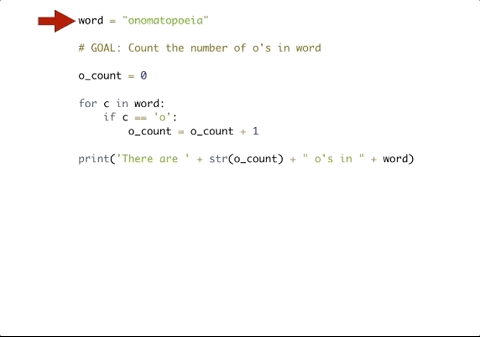# 10.18. The Accumulator Pattern with Lists¶

Remember the accumulator pattern? Many algorithms involving lists make use of this pattern to process the items in a list and compute a result. In this section, we’ll explore the use of the accumulator pattern with lists.

Let’s take the problem of adding up all of the items in a list. The following program computes the sum of a list of numbers.

The program begins by defining an accumulator variable, `sum`, and initializing it to 0 (line 1).

Next, the program iterates over the list (lines 2-3), and updates the sum on each iteration by adding an item from the list (line 3). When the loop is finished, `sum` has accumulated the sum of all of the items in the list.

Take a moment to step through this program using CodeLens to see how it works. It’s important to grasp the basic techniques.

Sometimes when we’re accumulating, we don’t want to add to our accumulator every time we iterate. Consider, for example, the following program which counts the number of names with more than 3 letters.

Here, we initialize the accumulator variable to be zero on line 1.

We iterate through the sequence (line 2).

The update step happens in two parts. First, we check to see if the name is longer than 3 letters. If so, then we increment the accumulator variable `long_names` (on line 4) by adding one to it.

At the end, we have accumulated the total number of long names.

We can use conditionals to also count if particular items are in a string or list. The following code finds all occurrences of vowels in a string.

We can also use `==` to execute a similar operation. Here, we’ll check to see if the character we are iterating over is an “o”. If it is an “o” then we will update our counter.## 10.18.1. Accumulating the Max Value¶

We can also use the accumulation pattern with conditionals to find the maximum or minimum value. Instead of continuing to build up the accumulator value like we have when counting or finding a sum, we can reassign the accumulator variable to a different value.

The following example shows how we can get the maximum value from a list of integers.

Here, we initialize best_num to zero, assuming that there are no negative numbers in the list.

In the for loop, we check to see if the current value of n is greater than the current value of `best_num`. If it is, then we want to update `best_num` so that it now is assigned the higher number. Otherwise, we do nothing and continue the for loop.

You may notice that the current structure could be a problem. If the numbers were all negative what would happen to our code? What if we were looking for the smallest number but we initialized `best_num` with zero? To get around this issue, we can initialize the accumulator variable using one of the numbers in the list.

The only thing we changed was the value of `best_num` on line 2 so that the value of `best_num` is the first element in `nums`, but the result is still the same!

## 10.18.2. Accumulating a String Result¶

The accumulator pattern can be used to convert a list of items to a string.

Consider the following program:

Here, the accumulator variable is `result`. Each time through the loop, the program concatenates the current contents of `result` with the comma separator and a score from the list, and updates the `result` with the new value. Use CodeLens to step through this example to see it in action.

The output of the program has some undesirable formatting problems: there is a trailing comma instead of a period, and there are no spaces between the items. The next activity lets you work to correct those problems.

Let’s work to improve the formatting of the sentence produced by the program above. Revise the following code so that it outputs the sentence:

```The scores are 85, 95, and 70.
```

Try changing the loop so that it does not process the final score. Handle that last score separately, after the loop finishes.

This solution works by iterating over all of the scores in the list except the last, and dealing with that one separately.

Challenge For each word in `words`, add ‘d’ to the end of the word if the word ends in “e” to make it past tense. Otherwise, add ‘ed’ to make it past tense. Save these past tense words to a list called `past_tense`.